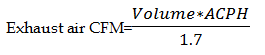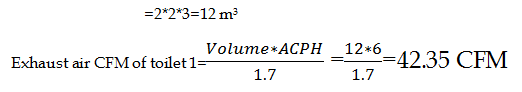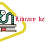# Toilet Ventilation

## Toilet Ventilation:

• Fans can either be installed directly in windows, walls or installed in air ducts system for exhausting and supplying the air into the toilet.
• In bathroom, toilet, kitchen areas negative pressure is maintained where usually supply air is 80% of the exhaust air.

Note:

As per ASHARE ,

1) Supply air 80% of the total exhaust air

2) For passing area, 1 square Feet= 1 CFM

Example:

Calculate the exhaust flow rate in public continue toilet?

Method 1:

As per ASHARE, 1 public continuous toilet CFM=50 CFM

10 public continuous toilet CFM=10*50=500 CFM

Passing area=10 m*2 m=20 m2=20*10.76=215.2 sq ft

As per ASHARE, For passing area 1 square feet = 1 CFM

Hence Passing area =215.2sq ft=215.2 CFM

Total Exhaust CFM=500+215.2=715.2 CFM

Fresh air cfm=715.2*0.8=572.16 CFM

Method 2(ACPH method):ACPH=6 to 10 (From data table)

Suppose ACPH=6

Volume of  Toilet 1=Length*width*HeightAll 10 toilet exhaust air cfm=10*42.35=423.5 CFM

Passing area=10 m*2 m=20 m2=20*10.76=215.2 sq ft

As per ASHARE, For passing area 1 square feet = 1 CFM

Hence Passing area =215.2sq ft=215.2 CFM

Total Exhaust air CFM=423.5+215.2=638.7 CFM

Fresh air CFM=0.8*638.7=510.96 CFM

1.2.